Divisor Drips andSquare Root Waves Prime Numbers are the Holes in Complex Composite Number Patterns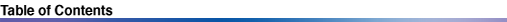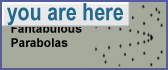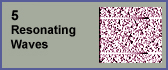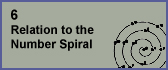4. Fantabulous Parabolas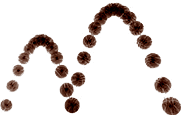Parabolas appear often in the physical world, and also in mathematical visualizations, such as what we have just seen in the previous chapter. Parabolas appear when you plot a graph of the subdivision of sine waves into integer-number frequencies, like the harmonics in a guitar string, vibrating in halves, thirds, quarters, fifths, and so-on.A big surprise came when I started building the divisor plot on a computer, and could see the divisors of larger numbers. Looking closely at the square root boundary, I started to make out a series of parabolas, by connecting nearby dots. These parabolas are kind of like the cross-sections of compressed, folded pieces of lettuce in a Dagwood sandwich ... or the curved edges of a folded blanket.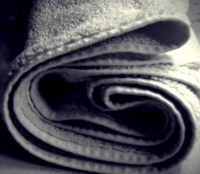Parabolas in the divisor plot represent a special view into the world of composite numbers. All parabola vertices point from left to right (from smaller numbers to larger numbers). Upon first discovering these parabolas, one gets the intuitive sense that something interesting might be going at in these locations. It is almost as if an infinite series of fingers along the square root boundary are saying, "look here, look here!"So let's look at these "square root parabolas". They are infinitely nested, and their vertices run along the square root boundary. Below is an example of the square root parabola located at x=100, and y=10 (the square root of 100).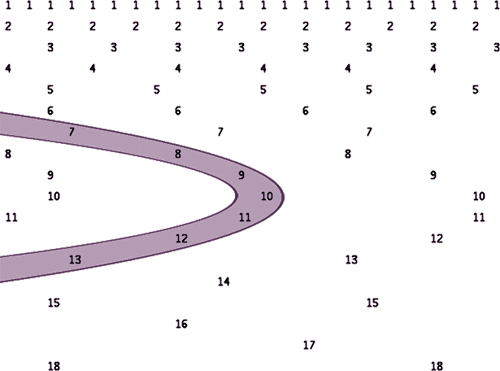I will refer to the entire set of square root parabolas as P, and I will refer to individual parabolas with the value n, an integer that can range from 1 to infinity. Here is an equation that I came up with to describe the curve of any square root parabola Pn: x = (n/2)2 - (y - n/2 )2 In this equation, y ranges from 0 to n. Consider this as an exponential Diophantine equation (an algebraic curve that uses only integers). It defines a set of lattice points that correspond to sets of divisors, numbered 1 to n, as shown below. It appears as if the entire set P fully tessellates the divisor plot: it intersects every divisor. Every divisor pair is a member of one and only one square root parabola. This is illustrated in the image below showing P1 through P8, indicated with straight lines connecting the ordinal series of divisors that lie on them.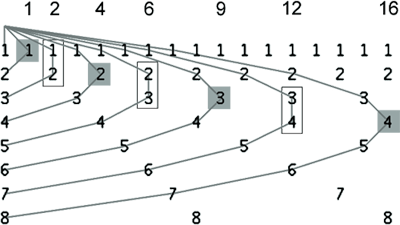The arms of each parabola Pn stretch out to the left, intersecting the zero divisor drip at (0,0) and (0, n). Notice how each even-numbered Pn has a perfect square root divisor located at its vertex. Each odd-numbered Pn, on the other hand, does not have a single divisor but instead has a pair of divisors slightly to the left of where the vertex would lie. These two divisors have a difference of 1. That means their product is a pronic number: a number that has two divisors with a difference of one. Thus, the first three pronics, (2, 6, and 12), are represented here (not including 0, which can be expressed as 0 x 1). All Pn have the same overall parabola shape. But due to their x locations on the lattice, even and odd-numbered Pn exhibit different spacing in x between their divisors, as shown here.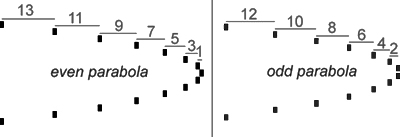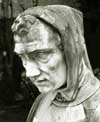An Observation about Square Numbers Fibonacci observed that square numbers can be constructed as sums of consecutive odd numbers. Look again at the even-numbered parabola I just showed you. The x-spacing between divisor pairs corresponds to the series of odd numbers, counting from right to left. Suppose that the vertex of this parabola were located at (100, 10). If you work leftwards from the vertex, and add the odd numbers shown, you get a series of squares: 1, 4, 9, 16, 25, 36, etc. Now, subtract each of these square numbers from 100. Subtract 1 from 100, and you get 99, which is the x value for the pair immediately to the left of the vertex. Subtract 4, and you get 96, which is the x value for the pair immediately to the left of that. Subtract 9 and you get 91... And so-on. Subtracting each of these square numbers from the x value of the vertex results in the x values for each pair of divisors on the parabola. Since we know that P represents a set of composite numbers, we arrive at the following general conclusion: Take any square number x, and then take each square number less than x and subtract it from x. All the numbers in this set (but not necessarily the lowest) are composite. Constant Sums Rob Sacks (whose number spiral we will visit soon ) observed that all divisor pairs in a square root parabola Pn have the same sum. This was a creative leap: instead of focusing on multiplication, what about addition? Not only did he observe that the divisors have the same sum, but in fact the sums are all equal to the parabola's index, n. This leads to one definition of a square root parabola: "a square root parabola Pn is the curve which intersects all divisor pairs in the divisor plot whose sums equal n." I tested to see if any other divisor pairs besides those on the parabola have the same sum, and it appears that there are none. And so, my hypothesis is that a square root parabola contains the only divisor pairs with this constant sum. Therefore, a square root parabola Pn can be defined more simply as: "the set of conjugate divisors in the divisor plot whose sum is n". One test I did is illustrated below, with parabola P48, whose vertex is located at (576, 24). The illustration highlights numerals for the divisor pairs whose sums equal 96 (which is 48 x 2). The image is greatly stretched vertically to make it easier to see.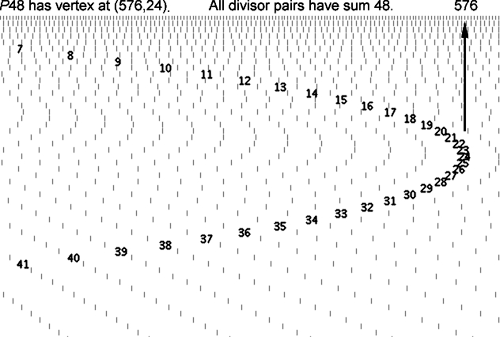Properties of Divisor Pairs on Square Root Parabolas The illustration below shows divisors near the vertex of P128, located at (4096, 64). Notice how divisor pairs to the left of the vertex converge towards the square root boundary as they get closer, and that there are no divisor pairs immediately to the right. We will confront this mystery later on.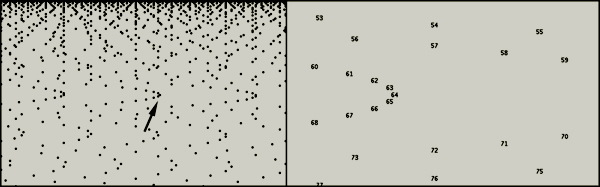The illustration below shows P2 through P8. Below them are symbols representing the products of the divisor pairs on these parabolas.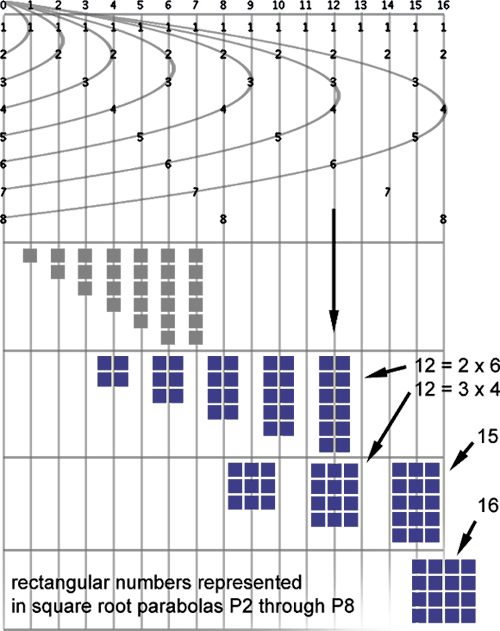Each symbol in this illustration is a rectangular array of blocks, where the number of blocks is equal to the product. These symbols illustrate the idea of a rectangular number. The number of columns in the array equals the divisor on the top arm of the parabola, and the number of rows equals the divisor on the bottom arm of the parabola. The number of columns and rows is equal in the case of square numbers. For example, the rectangular number 12 is shown as occurring on P7 and P8. The associated symbols show two of the three ways to express 12 (2x6 and 3x4). (The third way, 1x12, occurs on P13, which is not included in this illustration). This illustration may help give insight as to why these parabolas exist. The answer may have to do with the possible ways to arrange these blocks into rectangular arrays. Note that primes can only be represented in single-column, and that their divisors are always the second pair occurring at the left edge of a parabola (after the pair occurring at x=0). Approaching Squareness at Infinity As we observe the regions near the vertices of Pn at higher n, we see that their divisor pairs converge towards a ratio of 1. Since all Pn have the same shape, divisor pairs of numbers which are one-less than perfect squares converge towards "squareness", as n increases. For instance, compare the numbers 35 and 18,768, both of which are followed by a square number. Their closest divisors pairs are (5,7), and (136,138), respectively, as shown below. Even though each pair has a difference of 2, the ratios of those pairs are 0.714 and 0.985. Another way to express this is to refer to the rectangular arrays in the previous illustration. The rectangular arrays representing these pairs will appear more square in proportion at higher n.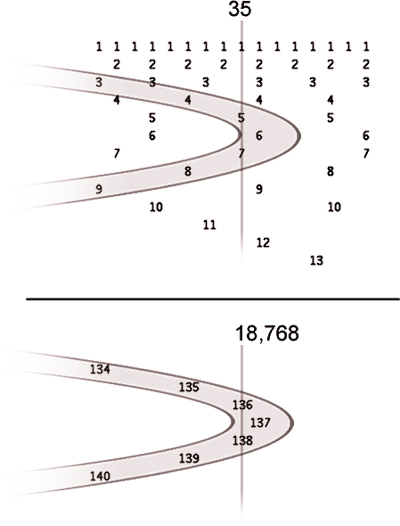As an oak grows from a tiny sapling to a giant adult tree, the roots sink deeper into the soil and become longer. This is similar to what happens in the divisor plot. As n increases, the square root parabola series sinks lower and lower into the soil of divisors.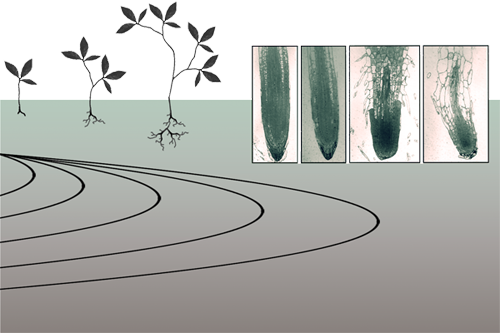But trees have many many roots. And alas, so does the divisor plot: the square root boundary is not the only parabola-rich place in the divisor plot. As we delve deeper and rightward along the divisor plot, the roots accumulate in number and they sink deeper.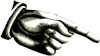Those "look here" fingers we saw earlier are pointing all over the place. In the next chapter, we will look at many varieties of parabolas, and try to get a better sense of why they exist.